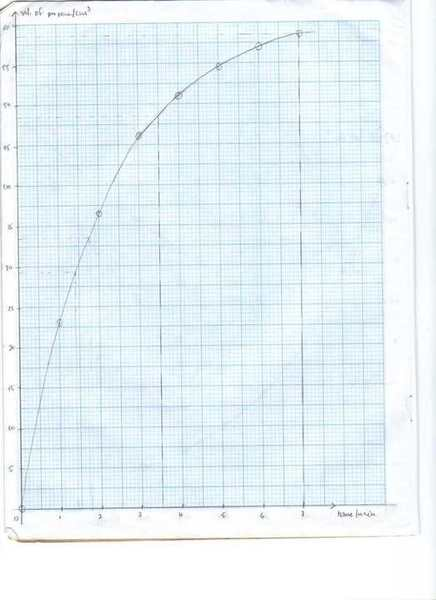# Kinetics/reaction rate

Kushal

## Homework Statement

cyclopropane isomerises to propene at a temperature of 500$$\circ$$C. The kinetics of the reaction was studied by measuring the volume of propene formed at every minute. The results obtained were tabulated below:

Plot these experiemntal results graphically.Use your graph to explain how the experimental results justify that the isomerisation is a fisrt order reaction.

## The Attempt at a Solution

ok, i know that if the reaction is first order, the half life of the graph should remain constant.

the problem is that the graph is about the volume of propene formed and not of the volume of cyclopropane isomerising.

how should i verify that this reaction is first order?